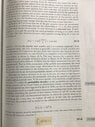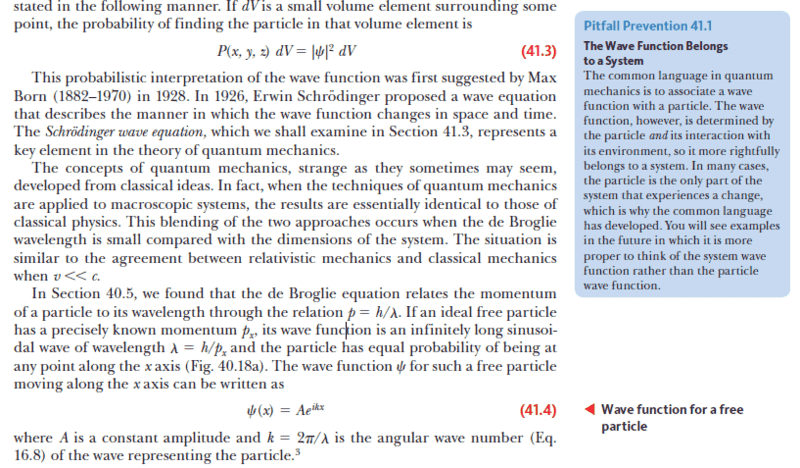# Understanding Wave Function Probabilities for a Free Particle

• I
• jjson775
The modulus is constant if and only if the modulus squared is constant.In my simplified case the modulus is not a constant (varies with x) but the modulus squared is a constant, so does that mean...In summary, the textbook I am self studying says that the wave function for a free particle with a known momentum, on the x axis, can be given as Asin(kx) and that the particle has an equal probability of being at any point along the x axis. I understand the square of the wave function to be the probability of finding the particle at a particular location. The square of Asin (kx) is not a constant so how can the probability be the same for allf

#### jjson775

TL;DR Summary
Location of a free particle with known momentum
The textbook I am self studying says that the wave function for a free particle with a known momentum, on the x axis, can be given as Asin(kx) and that the particle has an equal probability of being at any point along the x axis. I understand the square of the wave function to be the probability of finding the particle at a particular location. The square of Asin (kx) is not a constant so how can the probability be the same for all values of x? What am I missing?

The textbook I am self studying
Which textbook?

The textbook I am self studying says that the wave function for a free particle with a known momentum, on the x axis, can be given as Asin(kx)
This is not correct. The correct function is ##\exp(i k x)##. The probability (more precisely the probability density) is the squared modulus of this complex function times an appropriate normalization factor, which is of course a constant, independent of ##x##.

If you can give the specific textbook and the specific chapter/page reference, we can check to see whether the textbook itself is wrong or whether you are misinterpreting something it said.

•vanhees71 and malawi_glenn
Summary: Location of a free particle with known momentum

The textbook I am self studying says that the wave function for a free particle with a known momentum, on the x axis, can be given as Asin(kx) and that the particle has an equal probability of being at any point along the x axis. I understand the square of the wave function to be the probability of finding the particle at a particular location. The square of Asin (kx) is not a constant so how can the probability be the same for all values of x? What am I missing?
Moreover, such a wave-function is not physically realizable. The wave-function cannot be normalized.

Also, the modulus squared of the wave-function gives the probability distribution for the result of a measurement of position. It is not a probability distribution for where the particle is. A particle isn't anywhere until it is measured.

•vanhees71 and malawi_glenn
This is not correct. The correct function is ##\exp(i k x)##. The probability (more precisely the probability density) is the squared modulus of this complex function times an appropriate normalization factor, which is of course a constant, independent of ##x##.

If you can give the specific textbook and the specific chapter/page reference, we can check to see whether the textbook itself is wrong or whether you are misinterpreting something it said.

Serway Beichner Physics for Scientists and engineers, 5th edition, 2000, p. 1331.
See photo. An old book but I am old, too, an 80 year old retired engineer loving modern physics.

#### Attachments

•CC168AE4-54EC-4E4F-9112-85CB7BF30D40.jpeg
69.8 KB · Views: 51
••Demystifier, vanhees71 and PeroK
Serway Beichner Physics for Scientists and engineers, 5th edition, 2000, p. 1331.
See photo. An old book but I am old, too, an 80 year old retired engineer loving modern physics.
The author does seem to have got confused between de Broglie waves and wave-functions (i.e. solutions to the Schrodinger equation). And, in particular, ##\psi(x) = A \sin(kx)## does not have the property he claims of a constant modulus squared.

PS the author seems to be a little half-hearted about QM. It may be a minor point, but if you really commit to a QM view of nature, then you stop saying "where the particle is" and start saying "the result of a measurement of the particle's position". Failing to make this linguistic change shows a luke-warm approach to QM, IMHO.

I understand your valid point. Maybe the wording changed in a later edition.In the eight edition of 2010, the whole section is rewritten and corrected (see 41.4 in the capture from page 1221).

•Demystifier, vanhees71, malawi_glenn and 1 other person
Just forget this non-sensical book!

It’s not just the book. I need to learn about complex conjugates.

•PeroK

After some cleanup of a sub-thread about an error in a post, the thread is reopened. Thanks for your patience.•malawi_glenn
Technically it should be ##|A|^2## unless you assume that ##A## is a real number.

•vanhees71
Technically it should be ##|A|^2## unless you assume that ##A## is a real number.
Thanks. At my level of understanding, that was my assumption.

Thanks. At my level of understanding, that was my assumption.
Note also that a function with a constant modulus is not a viable (physically realisable) wavefunction. That's an important point.

•vanhees71
Note also that a function with a constant modulus is not a viable (physically realisable) wavefunction. That's an important point.
Do you mean the modulus squared?

•vanhees71
Do you mean the modulus squared?
The modulus is constant if and only if the modulus squared is constant.

•vanhees71
In my simplified case the modulus is not a constant (varies with x) but the modulus squared is a constant, so does that mean the wave function is viable?

In my simplified case the modulus is not a constant
Yes, it is. Only the phase (the ##\exp (i k x)## part) varies with ##x##.

•vanhees71
In my simplified case the modulus is not a constant (varies with x) but the modulus squared is a constant, so does that mean the wave function is viable?
The modulus of your function is ##|A|## and the modulus squared is ##|A|^2##. Both are constant.

Yes, it is. Only the phase (the ##\exp (i k x)## part) varies with ##x##.
I‘m not familiar with this terminology but am familiar with the sinusoidal wave equation and the Euler formula. If the exp term is the phase part, what do you call “A” in the wave function?

The modulus of your function is ##|A|## and the modulus squared is ##|A|^2##. Both are constant.
I would like to test my understanding of these points:

1. The modulus (absolute value) of the wave function is irrelevant except in terms of being the square root of the modulus squared.
2. in this simple case, the wave function is not physically viable because it does not meet the normalization condition.

#### Attachments

If the exp term is the phase part, what do you call “A” in the wave function?
Either the "amplitude" or the "modulus".

Got it. Thanks